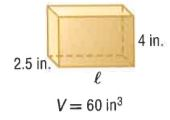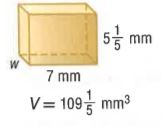Dear guest, you are not a registered member. As a guest, you only have read-only access to our books, tests and other practice materials.

As a registered member you can:

Registration is free and doesn't require any type of payment information. Click here to Register.
Volume and Surface Area - Test 1
Keywords: Common Core, Volume and Surface Area
• Question 1

Find the missing dimension of a rectangular prism with a volume of 126 cubic centimeters, a width of $$7\frac{7}{8}$$ centimeters, and height of 2 centimeters.

•  centimeters
• Question 2

A fishing tackle box is 13 inches long, 6 inches wide, and $$2\frac{1}{2}$$ inches high.
What is the volume of the tackle box?

•  V = in.³
• Question 3

Find the length of a rectangular prism having a volume of 2,830.5 cubic meters, width of 18.5 meters, and height of 9 meters.

•  l = meters

Find the missing dimension of each prism.

• Question 4•  $$l=$$ in.
• Question 5•  w = mm
• Question 6

In Japan, farmers have created watermelons in the shape of rectangular prisms. Find the volume of a prism -shaped watermelon in cubic inches if its length is 10 inches, its width is $$\frac{2}{3}$$foot, and its height is 9 inches.

•  V = cubic inches

Yes, email page to my online tutor.